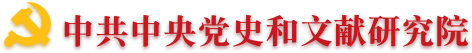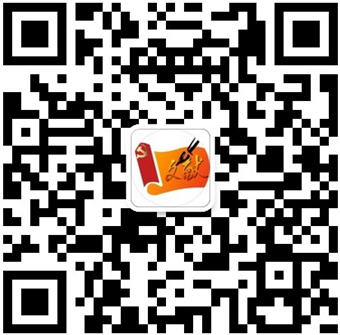•• 理論中國網   |
•"黨的文獻"公眾號"黃城根下"公眾號"黨的歷史"公眾號
•（微博）黨史網
“治平尚德行，有事賞功能”——從曹操的用人之道談“惟才是舉”與“以德為主”的統一

“治平尚德行，有事賞功能”

——從曹操的用人之道談“惟才是舉”與“以德為主”的統一

“治平尚德行，有事賞功能”，全面反映了曹操具體情況具體分析、靈活機動的用人之道，能夠更好地解釋曹操的用人實踐。

[作者孫業禮，中共中央文獻研究室編審，北京 100017]

（來源：《黨的文獻》2006年第3期）

### 專家學者

• 翻譯
• C
• D
• F
• G
• H
• J
• L
• M
• O
• P
• Q
• S
• T
• W
• X
• Y
• Z
• C
• F
• G
• H
• J
• L
• P
• Q
• S
• T
• W
• X
• Y
• Z
• C
• D
• G
• H
• J
• L
• Q
• S
• W
• X
• Y
• Z